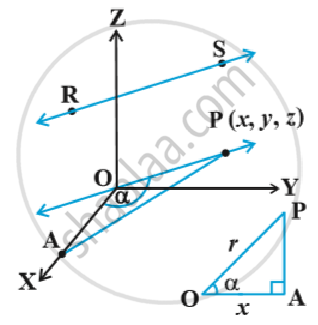# Relation Between Direction Ratio and Direction Cosines

#### notes

Consider a line RS with direction cosines l, m, n. Through the origin draw a line parallel to the given line and take a point P(x, y, z) on this line. From P draw a perpendicular PA on the x-axis in following Fig.Let OP = r. Then cos α = (OA)/(OP) = x / r .  This gives x = lr.
Similarly, y = mr and z = nr
Thus x^2 + y^2 + z^2 = r^2 (l^2 + m^2 + n^2)

But x^2 + y^2 + z^2 = r^2

Hence l^2 + m^2 + n^2 = 1